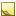﻿Angle data typeAngle data type

The data type Angle represents an angle measurement in a way that is unit-neutral.

The TDE data types for quantifiable data (numeric values with units of measure) are all stored as double and are handled similarly for template scripting.Tip

Since every quantifiable data type is stored as double, be sure to account for that when you test equivalency (for example, 1 may actually be stored as 0.999999). Either round off the values being compared, or use ranges for the comparison.Units
• Degree

• DDDMMSS — (output only) formats as degrees, minutes, seconds

Data from TDE defaults to the first unit listed for each data type. In the [Output] section, you can specify the degrees to use this formatting:

```[Output]
Latitude(DDDMMSS) = input.Lat```Field length

The range of this data type is to 13 decimals of precision.JavaScript conversion

When you use the optional [Script] section to insert your own JavaScript functions, access this data type as follows:

Constructor
JavaScript
`new Angle({Degree:Value})`
Available fields for (get,set)

You can access the valid units of measure as fields:

• .Degree

• .DDDMMSS (output only)